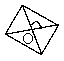The diagonals of a rhombus are 24 cm and 32 cm then its perimeter is

# The diagonals of a rhombus are 24 cm and 32 cm then its perimeter is

1. A
80 cm
2. B
60 cm
3. C
70 cm
4. D
none

Register to Get Free Mock Test and Study Material

+91

Live ClassesRecorded ClassesTest SeriesSelf Learning

Verify OTP Code (required)

### Solution:$4A{B}^{2}=A{C}^{2}+B{D}^{2}$

$⇒4A{B}^{2}={24}^{2}+{32}^{2}$

$⇒A{B}^{2}=\frac{576+1024}{4}$

$⇒A{B}^{2}=\frac{1600}{4}$

Perimeter (Rhombus) $=4×20$

$=80\text{\hspace{0.17em}}\text{cm}$

## Related content

 Distance Formula Perimeter of Rectangle Area of Square Area of Isosceles Triangle Pythagoras Theorem Triangle Formulae Volume of Cylinder Perimeter of Triangle Formula Area Formulae Volume Formulae